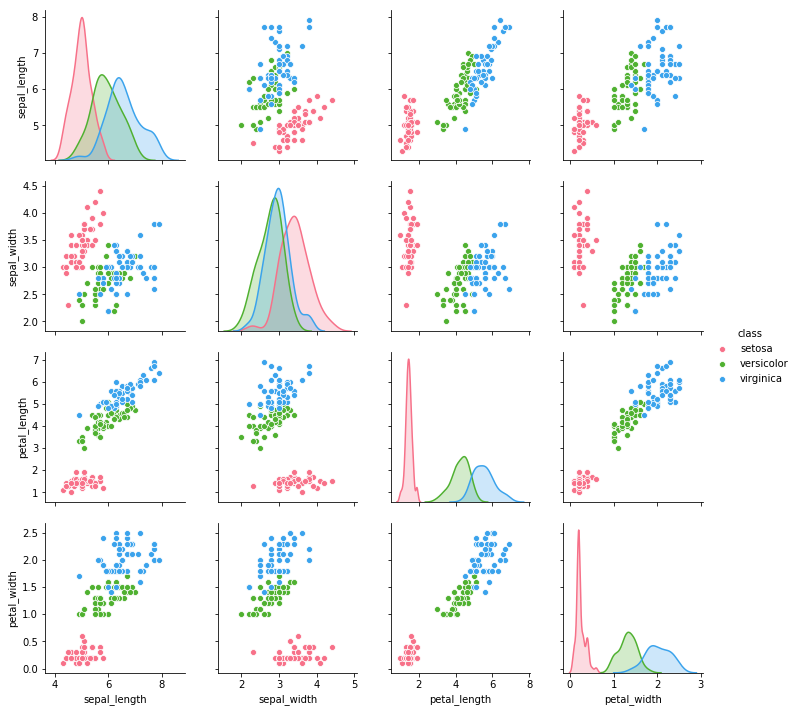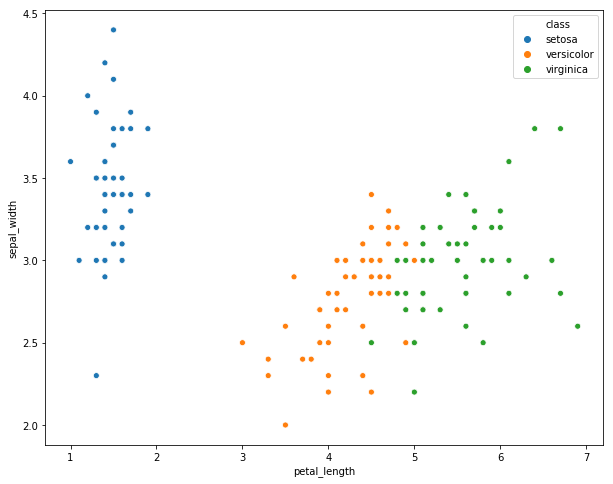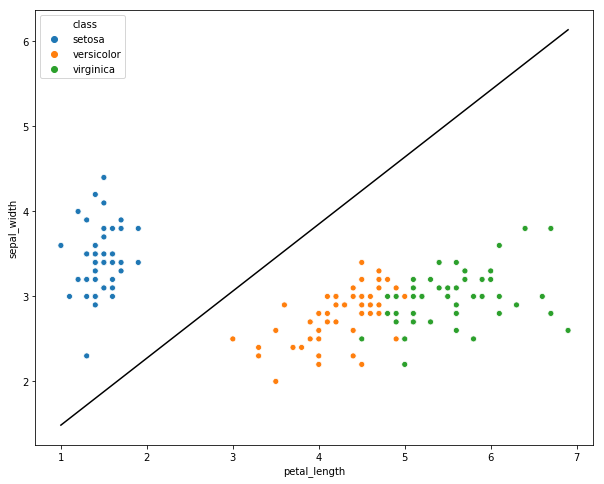# Jupyter Notebook Showcase¶

by Ian McLoughlin ([email protected])

This notebook demonstrates the analysis of a data set using the Python programming language.

The notebook is hosted on GitHub.

You can take this interactive notebook and play around with it: https://goo.gl/SbYMqr.

Note: unfortunately, Google haven't updated their packages yet so you'll have to uncomment and run the following code if you're running it on Collaboratory.

In :
#!pip install --upgrade seaborn


## A bit of context¶

#### My objectives in this talk are to:¶

1. Demonstrate that programming is accessible to non-computing students.
2. Discuss the pros and cons of Graphical User Interfaces in data analytics.
3. Widen understanding of the formal sciences.

#### A few talking points¶

• Notebooks are useful for students of all disciplines (formal sciences, natural sciences, social sciences, engineering, etc.)
• Notebooks are documents that blend text, mathematical notation and runnable code, and can be run from a browser.
• We'll soon have our first wave of incoming students having taken Computer Science at second level.
• That might foster a discussion about what programming is - maybe it's just a skill.

## About the data set¶We'll look at the well-known Iris data set.

It was collected by Ronald Fisher (above).

Fisher is famous for The Design of Experiments including the Lady Tasting Tea problem and ANOVA amongst other things.

## Load a data set¶Using Python, we can easily load a comma separated values (CSV) file to analyse it.

In :
# pandas is a Python package for investigating data sets.
import pandas as pd

# We can load a CSV file directly from a URL.
df = pd.read_csv("https://github.com/ianmcloughlin/datasets/raw/master/iris.csv")

# Have a look at the first five rows of the data set.
df.head(5)

Out:
sepal_length sepal_width petal_length petal_width class
0 5.1 3.5 1.4 0.2 setosa
1 4.9 3.0 1.4 0.2 setosa
2 4.7 3.2 1.3 0.2 setosa
3 4.6 3.1 1.5 0.2 setosa
4 5.0 3.6 1.4 0.2 setosa

## Plot the data¶

The data set contains five variables, and so is difficult to visualise. Luckily somebody has written a Python package called seaborn with a lovely plot suited to it.

In :
# Set up our Jupyer notebook to display plots nicely.
%matplotlib inline

In :
# Set the default plot size.
import matplotlib.pyplot as plt
plt.rcParams['figure.figsize'] = (10, 8)

In :
# seaborn is named after Rob Lowe's character in The West Wing.
import seaborn as sns

# A pair plot will create a matrix of scatter plots.
pp = sns.pairplot(df, hue="class", palette="husl")## Formulate a problem¶

Fisher was interested in knowing whether the class could be predicted from the other variables.

$$f(sl, sw, pl, pw) = class$$

Can we figure out a good $f$ from the data set?

## Try a simpler problem¶

The setosa class looks quite a bit different to the other two.

In :
# Let's single out two of the numeric variables.
sns.scatterplot(x="petal_length", y="sepal_width", hue="class", data=df)

Out:
<matplotlib.axes._subplots.AxesSubplot at 0x2899d16a198>In :
# Adapted from https://stackoverflow.com/questions/22491359/least-squares-linear-classifier-in-matlab
# Note the code here is a little more involved, but it can likely be simplified.

# Numerical package for Python
import numpy as np

# A will have three columns: petal lengths, sepal widths and a column of 1's.
A = df[['petal_length', 'sepal_width']].values
A = np.hstack([A, np.ones([A.shape, 1])])

# b is a column matrix that contains a 1 eveywhere there's a setosa and -1 elsewhere.
b = (df['class'] == 'setosa').map({True: 1, False: -1})

# Find the best x in Ax=b.
x = np.linalg.lstsq(A, b)

# Now we can plot the line on top of the previous plot.
sns.scatterplot(x="petal_length", y="sepal_width", hue="class", data=df)

u = np.array([min(df['petal_length']), max(df['petal_length'])])
v = -x/x - (x/x)*u;
plt.plot(u, v, 'k-')

C:\Users\mclou\Anaconda3\lib\site-packages\ipykernel_launcher.py:15: FutureWarning: rcond parameter will change to the default of machine precision times max(M, N) where M and N are the input matrix dimensions.
To use the future default and silence this warning we advise to pass rcond=None, to keep using the old, explicitly pass rcond=-1.
from ipykernel import kernelapp as app

Out:
[<matplotlib.lines.Line2D at 0x2899f18a6a0>]The idea is now that when you come across a new iris, you can decide whether it's a setosa or not by plotting its petal length and sepal width to see what side of the line it is on.

## Train a neural network¶

Using Python, we can get up and running with quite sophisticated concepts very quickly.For instance, we can create small neural network.

In :
# For building neural networks.
import keras as kr

# For encoding categorical variables.
import sklearn.preprocessing as pre

# For splitting into training and test sets.
import sklearn.model_selection as mod

inputs = df[['petal_length', 'petal_width', 'sepal_length', 'sepal_width']]

encoder = pre.LabelBinarizer()
encoder.fit(df['class'])
outputs = encoder.transform(df['class'])

# Start a neural network, building it by layers.
model = kr.models.Sequential()

# Add a hidden layer with 64 neurons and an input layer with 4.
model.add(kr.layers.Dense(units=64, activation='relu', input_dim=4))
# Add a three neuron output layer.
model.add(kr.layers.Dense(units=3, activation='softmax'))

# Build the graph.
model.compile(loss='categorical_crossentropy', optimizer='adam')

# Train the neural network.
model.fit(inputs, outputs, epochs=10, batch_size=5)

Using TensorFlow backend.

Epoch 1/10
150/150 [==============================] - 1s 5ms/step - loss: 0.8309
Epoch 2/10
150/150 [==============================] - 0s 327us/step - loss: 0.6564
Epoch 3/10
150/150 [==============================] - 0s 347us/step - loss: 0.5740
Epoch 4/10
150/150 [==============================] - 0s 480us/step - loss: 0.5263 0s - loss: 0.520
Epoch 5/10
150/150 [==============================] - 0s 500us/step - loss: 0.4801
Epoch 6/10
150/150 [==============================] - 0s 493us/step - loss: 0.4451
Epoch 7/10
150/150 [==============================] - 0s 347us/step - loss: 0.4241
Epoch 8/10
150/150 [==============================] - 0s 320us/step - loss: 0.4005
Epoch 9/10
150/150 [==============================] - 0s 440us/step - loss: 0.3717
Epoch 10/10
150/150 [==============================] - 0s 340us/step - loss: 0.3715

Out:
<keras.callbacks.History at 0x289a522fa90>
In :
# As an example, take the average values for a versicolor.
df[df['class'] == 'versicolor'].mean()

Out:
sepal_length    5.936
sepal_width     2.770
petal_length    4.260
petal_width     1.326
dtype: float64
In :
# Ask the neural network to predict what class of iris it is.
mean_versicolor = df[df['class'] == 'versicolor'].mean().values.reshape(1,4)
prediction = model.predict([mean_versicolor])
encoder.inverse_transform(prediction)

Out:
'virginica'

## A learning opportunity¶

You can see that the neural network predicts that the average versicolor to be a virginica.

When something like that happens, it's a good troubleshooting/learning opportunity for students.

You can let the students come up with ideas as to why the neural network thinks those values represent a virginica:

• Maybe the neural network is not set up correctly.
• Maybe taking the average of each variable does not represent the average versicolor.

## Concluding remarks¶

• Notebooks are useful for discussing and illuminating concepts.
• Python is an easy programming language to learn.
• We've touched on numerous different disciplines today.
• The processes, algorithms and techniques that enabled the analysis come largely from the formal sciences.